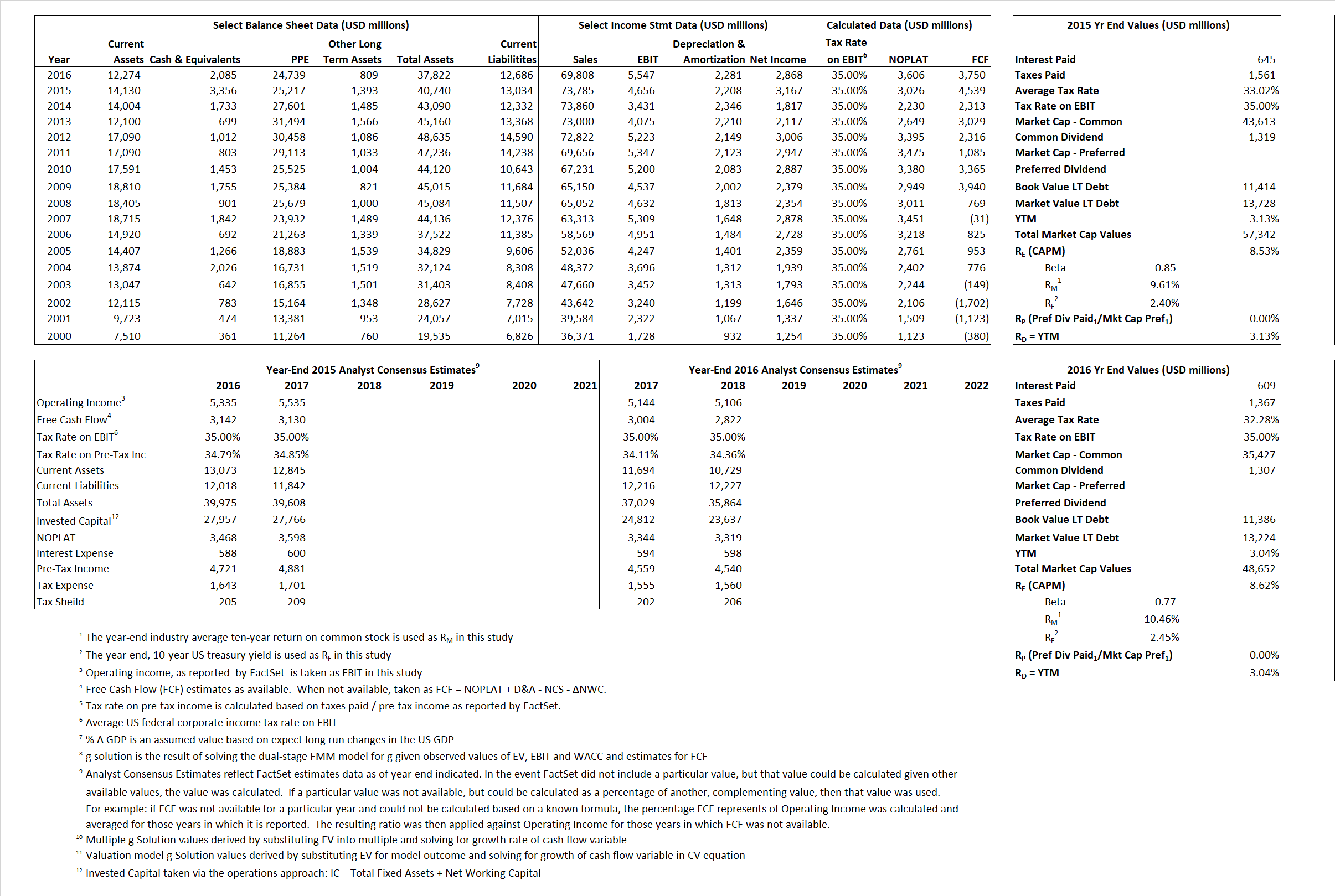# Target Corporation

## Analyst Listing

The following analysts provide coverage for the subject firm as of May 2016:

 Broker Analyst Analyst Email Jefferies Daniel Binder dbinder@jefferies.com Consumer Edge Research David A. Schick dschick@consumeredgeresearch.com Evercore ISI Greg Melich greg.melich@evercoreisi.com Cowen & Company Jason H. Seidl jason.seidl@cowen.com Buckingham Research John Zolidis jzolidis@buckresearch.com Telsey Advisory Group Joseph Feldman jfeldman@telseygroup.com Daiwa Securities Co. Ltd. Kahori Tamada kahori.tamada@us.daiwacm.com Credit Suisse Michael Exstein michael.exstein@credit-suisse.com Deutsche Bank Research Paul Trussell paul.trussell@db.com CRT Capital Group Renato Basanta rbasanta@sterneageecrt.com Nomura Research Robert S. Drbul robert.drbul@nomura.com Wolfe Research Scott Mushkin smushkin@wolferesearch.com Piper Jaffray Sean P. Naughton sean.p.naughton@pjc.com BMO Capital Markets Wayne Hood wayne.hood@bmo.com

## Primary Input Data## Derived Input Data

### Equational Form

Net Operating Profit Less Adjusted Taxes NOPLAT 3,026  3,606$NOPLAT\, =\, EBIT\, x\, (1 \,-\, Avg \,\,Tax\,\, Rate\,\, on\,\, EBIT)$
Free Cash Flow FCF 4,539 3,750$FCF\,=NOPLAT\,+\,Non-Cash\,Expenses-\Delta NWC\,-\,NCS$
Tax Shield TS 213 197$TS\,=\,Interest\,\,Paid\,\,x\,\, Avg \,\,Tax\,\,Rate\,\, on\,\, Pre-Tax\,\, Income$
Invested Capital IC 27,706 25,136$IC\,=\,Fixed\,\,Operating\,\,Assets\,\,+\,\,Net\,\, Working\,\, Capital$
Return on Invested Capital ROIC 10.92% 14.34%$ROIC\,=\,\frac { NOPLAT }{ IC }$
Net Investment NetInv (843) (289)$NetInv\,=\,{ {IC}_{1}}-{{IC}_{0}}+Depreciation$
Investment Rate IR  -27.87% -8.02%$IR\,=\,\frac {NetInv}{NOPLAT}$
Weighted Average Cost of Capital
WACCMarket 6.99% 6.83%$WACC\,=\,\frac { E }{ V } { R }_{ E }\,+\,\frac { P }{ V } { R }_{ P }\,+\,\frac { D }{ V } { R }_{ D }\left( 1- Avg\,\, Tax\,\,Rate\,\,on\,\,Pre-Tax\,\,Income \right)$
WACCBook   6.42%  6.17%
Enterprise value
EVMarket 53,986 46,567$EV\,=\,Market\,\,Cap\,\,Equity\,+\,\,Long\,\,Term\,\,Debt\,-\,Cash$
EVBook   52,942  44,728
Long-Run Growth
g = IR x ROIC
-3.04%  -1.15% Long-run growth rates of the income variable are used in the Continuing Value portion of the valuation models.
g = %$\Delta$ GDP   2.50%   2.50%
Margin from Operations M   6.31%  7.95%$M\,\,=\,\,\frac{EBIT}{SALES}$
Depreciation/Amortization Rate D   32.17%  29.14%$D\,\,=\,\,\frac{D+A}{EBITDA}$

## Valuation Multiple Outcomes

The outcomes presented in this study are the result of original input data, derived data, and synthesized inputs.

### model g solution

12/31/2015 12/31/2016 12/31/2015 12/31/2016 12/31/2015 12/31/2016

EV/SALES$\frac {EV}{Sales} \,= \,\frac{ROIC\, -\, g}{ROIC\,(WACC\,-\,g)}\,(1\,-\,T)\,(M)$

0.73  0.67  2.84%  -1.97%  3.04%  0.10%

EV/EBITDA$\frac {EV}{EBITDA} \,= \,\frac{ROIC\, -\, g}{ROIC\,(WACC\,-\,g)}\,(1\,-\,T)\,(1\,-\,D)$

7.87 5.95 2.84% -1.97% 3.04% 0.10%

EV/NOPLAT$\frac {EV}{NOPLAT} \,= \,\frac{ROIC\, -\, g}{ROIC\,(WACC\,-\,g)}$

17.84 12.92 2.84% -1.97% 3.04% 0.10%

EV/FCFOPS$\frac {EV}{FCF_{OPS}} \,= \,\frac{ROIC\, -\, g}{ROIC\,(WACC\,-\,g)}\,(1\,-\,T)$

11.89 12.42 2.84% -1.97% 3.04% 0.10%

EV/EBIT$\frac {EV}{EBIT} \,= \,\frac{ROIC\, -\, g}{ROIC\,(WACC\,-\,g)}\,(1\,-\,T)$

11.59 8.39 2.84% -1.97% 3.04% 0.10%

EV/IC$\frac {EV}{IC} \,= \,\frac{ROIC\, -\, g}{WACC\,-\,g}$

1.95 1.85 2.84% -1.97% 3.04% 0.10%